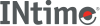﻿ qsort, qsort_sINtime SDK Help
qsort, qsort_s

Performs a quick sort of an array, overwriting the input array with the sorted elements.

```#include <stdlib.h>
#include <search.h>

void qsort (void *base, size_t num, size_t width,
int (*compare)(const void *elem1, const void *elem2));errno_t qsort_s (void *base, size_t num, size_t width,
int (*compare)(const void *elem1, const void *elem2, void *context),            void *context);
```

#### Parameters

`base`
Pointer to the base of the array you want to sort and overwrite.
`num`
Array size in number of elements.
`width`
Element size in bytes.
`compare`
Pointer to a user-supplied routine that compares two array elements (`elem1` and `elem2`) and returns a value specifying their relationship:
 `< 0` `elem1` less than `elem2` `= 0` `elem1` equivalent to `elem2` `> 0` `elem1` greater than `elem2`
`elem1`
Pointer to the key for the sort.
`elem2`
Pointer to the array element to compare with the key.

#### Remarks

qsort calls the compare routine one or more times during the sort, passing pointers to two array elements on each call:

```compare (( void *) elem1, (void *) elem2);
```

The function sorts the array in ascending order, as defined by the compare routine. To sort the array in descending order, reverse the sense of greater-than and less-than in the compare routine.

#### Description

The qsort() function is a modified partition-exchange sort, or quicksort.

The qsort() function sorts an array of nmemb objects, the initial member of which is pointed to by base. The size of each object is specified by size.

The contents of the array base are sorted in ascending order according to a comparison function pointed to by compar, which requires two arguments pointing to the objects being compared.

The comparison function must return an integer less than, equal to, or greater than zero if the first argument is considered to be respectively less than, equal to, or greater than the second.

The algorithms implemented by qsort() are not stable, that is, if two members compare as equal, their order in the sorted array is undefined.

The qsort() function is an implementation of C.A.R. Hoare's "quicksort" algorithm, a variant of partition-exchange sorting; in particular, see D.E. Knuth's Algorithm Q. Quicksort takes O N lg N average time. This implementation uses median selection to avoid its O N**2 worst-case behavior.

The qsort_s() function behaves the same as qsort(), except that:

• The order of arguments is different
• The order of arguments to compare is different
• if num or width are greater than RSIZE_MAX, or num is not zero and compare is NULL, then the runtime-constraint handler is called, and qsort_s() returns an error. Note that the handler is called before qsort_s() returns the error, and the handler function might not return.

#### Requirements

Versions Defined in Include Link to
INtime 3.0 intime/rt/include/stdlib.h stdlib.h
search.h
clib.lib